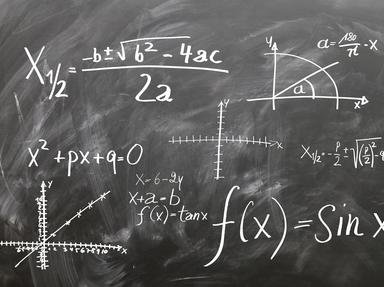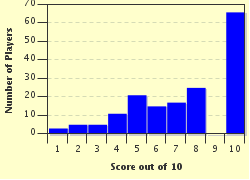# What's My Number? Trivia Quiz

### Can you find the number that matches each description?

A matching quiz by looney_tunes. Estimated time: 4 mins.

Author
looney_tunes
Time
4 mins
Type
Match Quiz
Quiz #
380,057
Updated
Dec 03 21
# Qns
10
Difficulty
Average
Avg Score
8 / 10
Plays
780
Awards
Top 35% Quiz
Last 3 plays: Guest 188 (10/10), Guest 122 (10/10), Guest 86 (2/10).
Mobile instructions: Press on an answer on the right. Then, press on the gray box it matches on the left.
(a) Drag-and-drop from the right to the left, or (b) click on a right side answer box and then on a left side box to move it.
 Questions Choices 1. The only integer which is neither positive nor negative. 4 2. The smallest perfect number. 15 3. Number of dimensions of a plane (mathematical, not aeronautical). 1 4. Number of faces on an icosahedron. 6 5. The fifth triangular number. 6 6. Number of faces on a cube. 2 7. Number of equal sides in an isosceles triangle. 8 8. Number of vertices on a cube. 0 9. The only positive integer which is neither prime nor composite. 20 10. Number of dimensions of a tesseract. 2

Quiz Answer Key and Fun Facts
1. The only integer which is neither positive nor negative.

A positive number is greater than zero, while a negative number is less than zero (which is indicated by writing it with a - before the digits that represent its magnitude). 0 itself is the only number which is neither greater than zero nor less than zero.
2. The smallest perfect number.

A perfect number is a positive integer which is the sum of all its proper divisors. Clear as mud? The divisors are the numbers that can divide evenly into a given number, and the proper divisors are smaller than the original number. The divisors of 6 are 1, 2, 3 and 6 (since 6 = 1x6 and 6=2x3). The proper divisors are 1, 2 and 3. The sum of these three numbers is 1+2+3 = 6. This is the smallest number for which it works:
1 - no proper divisors
2 - 1
3 - 1
4 - 1+2 = 3
5 - 1
3. Number of dimensions of a plane (mathematical, not aeronautical).

A point has no dimensions. If a point is translated in a single direction, the result is a line, which has one dimension. If a line is translated in a direction perpendicular to its length, a plane is produced, which has two dimensions.
4. Number of faces on an icosahedron.

If your Greek is good, you should recognise the prefix used to describe a polyhedron with twenty sides. A regular icosahedron has equilateral triangles for its faces, with five triangles meeting at each of its twelve vertices.
5. The fifth triangular number.

Triangular numbers are a sequence which can be listed as 1, 3, 6, 10, 15, 21, ... - as you can see, each number is larger than the previous one by an amount which increases by 1 each time. Since this is a tedious procedure if you want to know, say, the 100th one, there is a formula which can be used to calculate it: Tn = n(n+1)/2.

The 100th triangular number is therefore 100x101/2, or 505.
6. Number of faces on a cube.

A cube is a three-dimensional figure made up of 6 squares - a standard die is probably the most familiar example. A cuboid is a similar shape, but with some or all of the faces being rectangles instead of squares - think of a ream of paper, for example.
7. Number of equal sides in an isosceles triangle.

A triangle with no equal sides is called a scalene triangle, while one with all three sides equal is an equilateral triangle. As well as names describing the relative lengths of a triangle's sides, they can also be given a name that tells you the size of the largest of the three angles: for an acute triangle, all angles are less than 90 degrees; for a right angled triangle, one angle is 90 degrees; for an obtuse angled triangle, one angle is between 90 and 180 degrees. Since the sum of the three angles in a triangle is always 180 degrees, no one angle can be as large as 180, let alone larger than that.
8. Number of vertices on a cube.

The vertices are often referred to as corners in everyday usage, but mathematicians prefer the technical term vertex to refer to a point where two or more faces of a polyhedron meet. For a cube, there are three square faces meeting at each vertex.
9. The only positive integer which is neither prime nor composite.

The definition of a prime number is that it is a number greater than one which is divisible only by itself and one. (This definition eliminates a number of mathematical paradoxes that would arise if 1 were included as a prime number.) A composite number is one with at least two proper divisors (two numbers smaller than itself by which it is divisible).

For example, 2 can only be divided by 1 and 2, so it is the smallest prime number; 4 can also be divided by 1 and 2, and is the smallest composite number.
10. Number of dimensions of a tesseract.

As described earlier a point can be translated to generate a line, which can be translated to generate a plane. If we only use a segment of the line, we can translate it so as to form a square. If that square is moved upwards, in a third dimension perpendicular to that of the original plane, we can produce a cube. Now, mathematically (if not physically), that same process can be repeated using a fourth dimension that is orthogonal to the cube, called a tesseract.
Source: Author looney_tunes

This quiz was reviewed by FunTrivia editor rossian before going online.
Any errors found in FunTrivia content are routinely corrected through our feedback system.
Most Recent Scores
Jan 23 2023 : Guest 188: 10/10
Jan 13 2023 : Guest 122: 10/10
Jan 05 2023 : Guest 86: 2/10
Dec 20 2022 : Vendetta125: 4/10
Dec 09 2022 : Guest 101: 6/10

Score DistributionRelated Quizzes
This quiz is part of series Maths Challenges:

A collection of quizzes aimed at players with a high school level of mathematics who are interested in exploring the world of mathematics.

1. Transcendental Numbers Average
2. Perfect and Not-So-Perfect Numbers Tough
3. The Matrix Average
4. Periodic Functions Difficult
5. Shapes From Squares Average
6. You Derive Me Crazy! Average
7. What's My Number? Average
8. What's My Type? Average